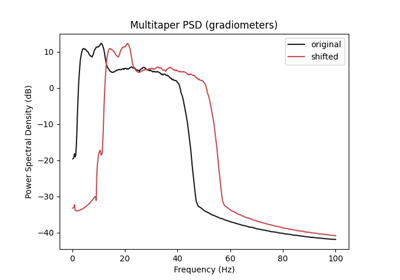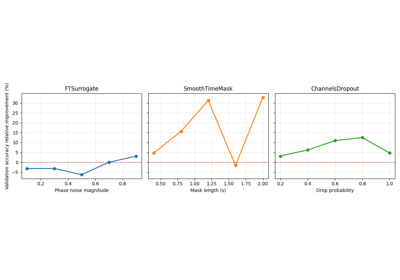# braindecode.augmentation.Transform#

class braindecode.augmentation.Transform(probability=1.0, random_state=None)[source]#

Basic transform class used for implementing data augmentation operations.

Parameters
• operation (callable) – A function taking arrays X, y (inputs and targets resp.) and other required arguments, and returning the transformed X and y.

• probability (float, optional) – Float between 0 and 1 defining the uniform probability of applying the operation. Set to 1.0 by default (e.g always apply the operation).

• random_state (int, optional) – Seed to be used to instatiate numpy random number generator instance. Used to decide whether or not to transform given the probability argument. Defaults to None.

Methods

forward(X: Tensor, y: = None) [source]#

General forward pass for an augmentation transform.

Parameters
• X (torch.Tensor) – EEG input example or batch.

• y (torch.Tensor | None) – EEG labels for the example or batch. Defaults to None.

Returns

• torch.Tensor – Transformed inputs.

• torch.Tensor, optional – Transformed labels. Only returned when y is not None.

get_augmentation_params(*batch)[source]#

## Examples using `braindecode.augmentation.Transform`#Data Augmentation on BCIC IV 2a Dataset

Data Augmentation on BCIC IV 2a DatasetSearching the best data augmentation on BCIC IV 2a Dataset

Searching the best data augmentation on BCIC IV 2a Dataset